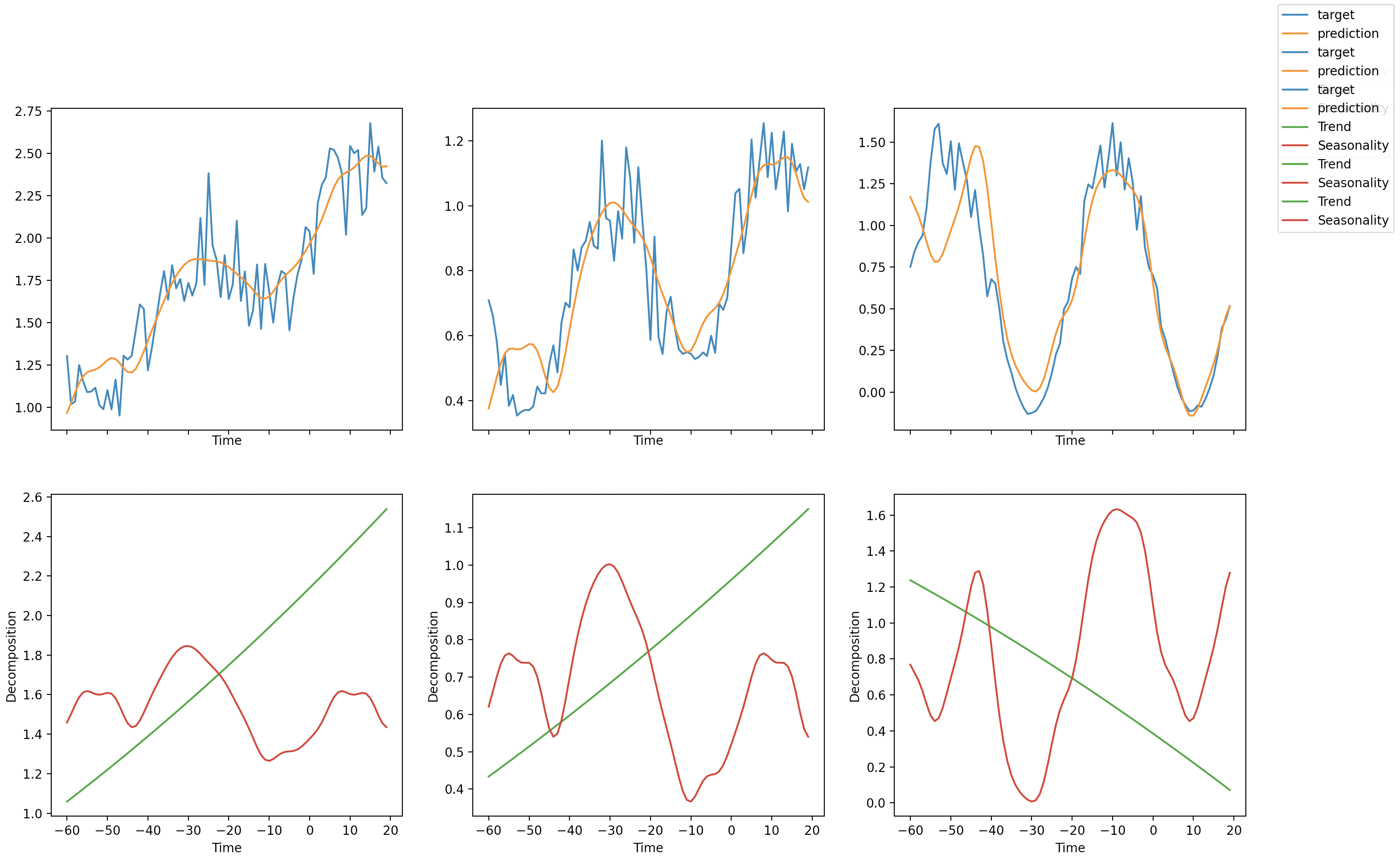Shortcuts

# PyTorch Forecasting¶

PyTorch Forecasting provides the models and data loading for the Tabular Forecasting task in Flash. As with all of our tasks, you won’t typically interact with the components from PyTorch Forecasting directly. However, PyTorch Forecasting provides some built-in plotting and analysis methods that are different for each model which cannot be used directly with the TabularForecaster. Instead, you can access the PyTorch Forecasting model object using the pytorch_forecasting_model attribute. In addition, we provide the convert_predictions() utility to convert predictions from the Flash format into the expected format. With these, you can train your model and perform inference using Flash but still make use of the plotting and analysis tools built in to PyTorch Forecasting.

Here’s an example, plotting the predictions and interpretation analysis from the NBeats model trained in the Tabular Forecasting documentation:

import torch

import flash
from flash.core.integrations.pytorch_forecasting import convert_predictions
from flash.core.utilities.imports import example_requires
from flash.tabular.forecasting import TabularForecaster, TabularForecastingData

example_requires(["tabular", "matplotlib"])

import matplotlib.pyplot as plt  # noqa: E402
import pandas as pd  # noqa: E402
from pytorch_forecasting.data import NaNLabelEncoder  # noqa: E402
from pytorch_forecasting.data.examples import generate_ar_data  # noqa: E402

# Example based on this tutorial: https://pytorch-forecasting.readthedocs.io/en/latest/tutorials/ar.html
# 1. Create the DataModule
data = generate_ar_data(seasonality=10.0, timesteps=400, n_series=100, seed=42)
data["date"] = pd.Timestamp("2020-01-01") + pd.to_timedelta(data.time_idx, "D")

max_prediction_length = 20

training_cutoff = data["time_idx"].max() - max_prediction_length

datamodule = TabularForecastingData.from_data_frame(
time_idx="time_idx",
target="value",
categorical_encoders={"series": NaNLabelEncoder().fit(data.series)},
group_ids=["series"],
# only unknown variable is "value" - and N-Beats can also not take any additional variables
time_varying_unknown_reals=["value"],
max_encoder_length=60,
max_prediction_length=max_prediction_length,
train_data_frame=data[lambda x: x.time_idx <= training_cutoff],
val_data_frame=data,
batch_size=32,
)

# 2. Build the task
model = TabularForecaster(
datamodule.parameters,
backbone="n_beats",
backbone_kwargs={"widths": [32, 512], "backcast_loss_ratio": 0.1},
)

# 3. Create the trainer and train the model
trainer = flash.Trainer(max_epochs=1, gpus=torch.cuda.device_count(), gradient_clip_val=0.01)
trainer.fit(model, datamodule=datamodule)

# 4. Generate predictions
datamodule = TabularForecastingData.from_data_frame(predict_data_frame=data, parameters=datamodule.parameters)
predictions = trainer.predict(model, datamodule=datamodule)
print(predictions)

# Plot with PyTorch Forecasting!
predictions, inputs = convert_predictions(predictions)

fig, axs = plt.subplots(2, 3, sharex="col")

for idx in range(3):
model.pytorch_forecasting_model.plot_interpretation(inputs, predictions, idx=idx, ax=[axs[idx], axs[idx]])

plt.show()


Here’s the visualization:© Copyright 2020-2021, PyTorch Lightning. Revision da42a635.

Built with Sphinx using a theme provided by Read the Docs.
Read the Docs v: stable
Versions
latest
stable
0.8.0
0.7.5
0.7.4
0.7.3
0.7.2
0.7.1
0.7.0
0.6.0
0.5.2
0.5.1
0.5.0
0.4.0
0.3.2
0.3.1
0.3.0
0.2.3
0.2.2
0.2.1
0.2.0
0.1.0post1